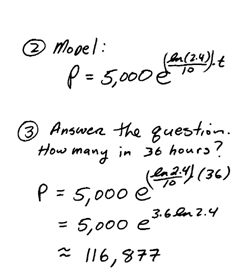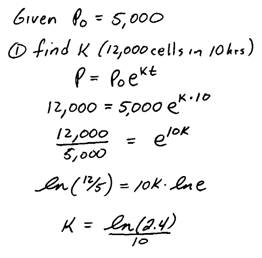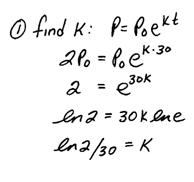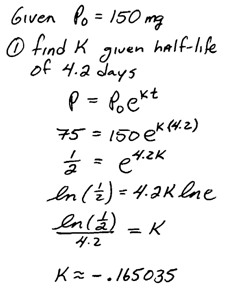## Algebra

### Exponential Growth and Decay

In this section we will solve typical word problems that involve exponential growth or decay.If k is positive then we will have a growth model and if k is negative then we will have a decay model.

Use the exponential growth/decay model to answer the questions.

Example: A certain bacterium has an exponential growth rate of 25% per day.  If we start with 0.5 gram and provide unlimited resources how much bacteria can we grow in 2 weeks?Answer: We can grow 16.56 grams of bacteria in 2 weeks time.

Typically the exponential growth rate will not be given.  In this case, we must determine that before we can use the model to answer the question.

Step 1: Use the given information to calculate the growth/decay rate k.
Step 2: Substitute the initial amount and k to formulate a model.
Step 3: Us the model to answer the question.

Example: During its exponential growth phase, a certain bacterium can grow from 5,000 cells to 12,000 cells in 10 hours.  At this rate how many cells will be present after 36 hours?Tip: Use the exact value for k and avoid round-off error.  If we use the approximate rounded-off value for k we will compound the error by rounding off again at the end when calculating the final result.

Example: During its exponential growth phase, a certain bacterium can grow from 5,000 cells to 12,000 cells in 10 hours.  At this rate how long will it take to grow to 50,000 cells?Answer: It will take approximately 26.3 hours.

Example: A certain animal species can double its population every 30 years. Assuming exponential growth, how long will it take the population to grow from 40 specimens to 500?Up to this point, we have seen only exponential growth.  We will conclude this section with some exponential decay applications.  Often exponential rate of decay can be determined from the half-life information.  Half-life is the amount of time it takes for a substance to decay to half of the original amount.

Example: A certain isotope has a half-life of 4.2 days. How long will it take a 150-milligram sample to decay so that only 10 milligrams are left?